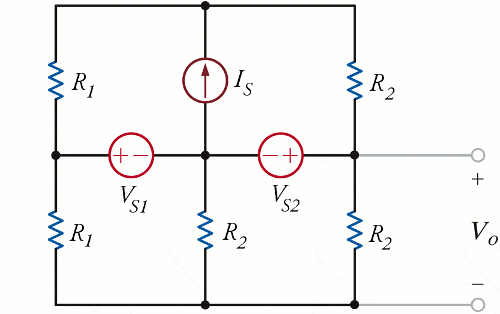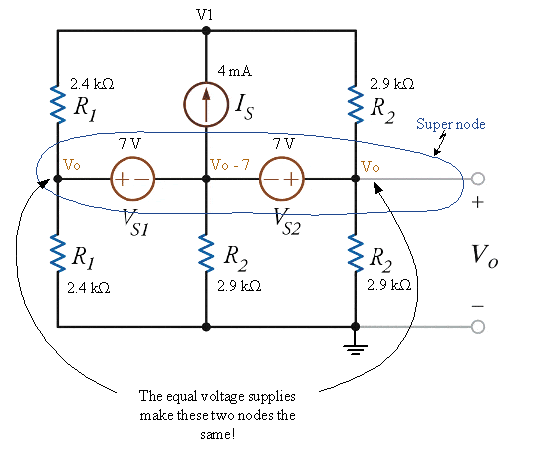# Thevenin Resistance problem

jgv115

## Homework StatementWhere R1 = 2.4k, R2 = 2.9k, Vs1=7V, Vs2=7v and Is = 4mA

For this circuit I need to find the Thevenin Equivalent resistance.

## The Attempt at a Solution

*Just a heads up, all my loop analysis stuff is going clockwise*

I've tried everything to try to solve this problem but I still can't get it. Here's what I've tried:

To find Rth I need Voc and Isc.

So to find Voc I used superposition:

If I short circuit Vs1 and Vs2, I get Vo(1) = 2.4k * 4mA = 9.6V

If I open circuit Is and short circuit Vs2 and use loop analysis:

2.4k(i1) + 7 +2.9k(i1) = 0
Solving for i1 = -0.001321A
Therefore Vo(2) = 2.9k * i1 = 3.83V

Then I open circuit Is and short circuit Vs1 wouldn't Vo(3) just be Vs2 = 7V?

Am I on the right track or have I completely gone off course? Any help is appreciated!

Mentor
The other way to find Vth is to suppress all the sources and find the equivalent resistance looking into the network at the output terminals; you don't have to determine the short circuit current.

jgv115
Hi gneill,

I've thought about doing that but because of the way the circuit is built, I don't quite understand which resistors are in series/parallel with other resistors.

Can you give me some tips/hints?

Gold Member
Notice that Vs1 and Vs2 "isolate" the top two loops from the bottom two loops.

Any current provided by Is flows only in Vs1 and Vs2; it can't reach the bottom resistors.

If you eliminate the top two resistors and Is, the behavior at the Vo terminals is unaffected; the Thevenin equivalent is the same with the top two loops gone, and that's an easier calculation.

Mentor
A hint: The voltage sources keep the potential differences between the middle three nodes at fixed values. In other words, what's called a supernode is formed by those nodes. As luck would have it the two voltage sources have the same value! And with the way they're oriented, the leftmost node must always have the same potential as the rightmost (Vo). So they are electrically the same node! There's your opportunity to not only put some resistors in parallel, but to eliminate one of the voltage supplies completely#### Attachments

Gold Member
gneill, may I ask what software you use to annotate the OP's image?

Mentor
gneill, may I ask what software you use to annotate the OP's image?

Sure. I use an old version of Visio. I copy/paste to Visio, mark it up, then copy/paste to Paint to save it as a gif. (Visio can create gifs directly, but it uses a filter of some sort that is tedious to use. With paint there are no options, just "Save as". Actually takes much less time!)